# The mathematics of optimization for machine learning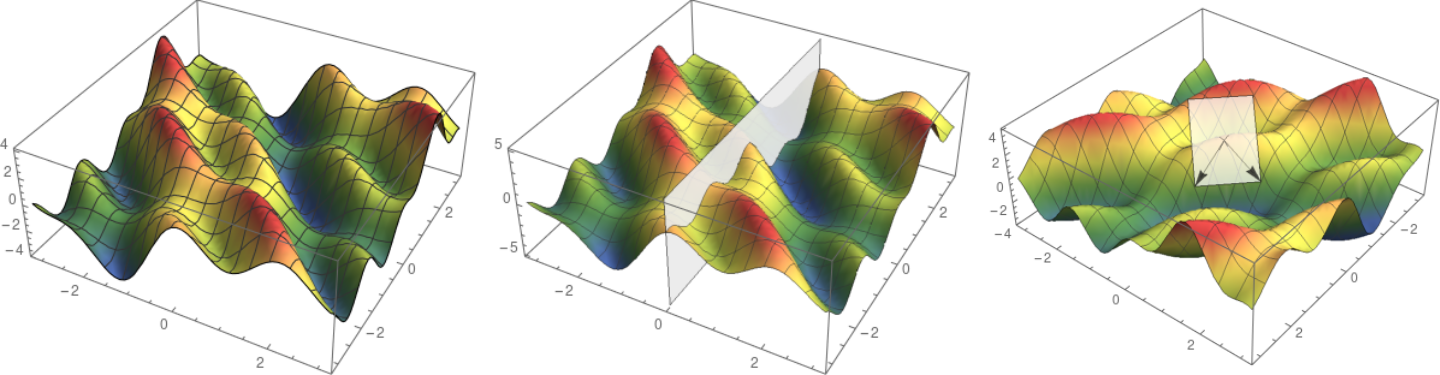In general, the overall performance of a neural network depends on several factors. The one usually taking the spotlight is the network architecture. However, this is only one among many essential components. An often overlooked contributor to a performant algorithm is the optimizer used to fit the model.

To illustrate the complexity of optimizing, consider a ResNet18 architecture that has 11689512 parameters. Finding an optimal parameter configuration is the same as locating a point in the 11689512-dimensional space. If we brute force this, we might divide this space up to a grid, selecting 10 points along each dimension. Then we have to check 10¹¹⁶⁸⁹⁵¹² possible configurations, calculate the loss function for each of them and find the one with minimal loss. To put this number in perspective, the observable universe has about 10⁸³ atoms, and it is estimated to be 4.32 x 10¹⁷ seconds (~13.7 billion years) old. If we check as many parameter configurations in each second as the number of atoms starting from the Big Bang, we would have been able to check 4.32 x 10¹⁴¹¹ points until now.

To say that this is not even close is an understatement. The grid size is still approximately 10⁸²⁸⁴ times larger than we could check if we would have every atom in the universe check a configuration since the Big Bang.

So, optimizers are pretty important. They manage this incomprehensible complexity, allowing you to train the neural networks in days instead of billions of years. In the following, we will take a deep dive into the mathematics of optimizers and see how they handle this seemingly impossible task.

## The basics of optimization

Let's start simple and suppose that we have a function of one variable which we would like to maximize. (In a machine learning context, we generally aim to minimize the loss function, but minimizing is the same as maximizing the negative of the function.) Definewhich looks like the following if we plot its graph.

An obvious method to optimize would be to divide the line into a grid, check the value of every point and select the one where the function is maximized. As we have seen in the introduction, this is not scalable. Thus, we must look for another solution.

Let's imagine that this is a mountain landscape, and we are climbers trying to reach the peak. Suppose that we are at the location marked with a red dot.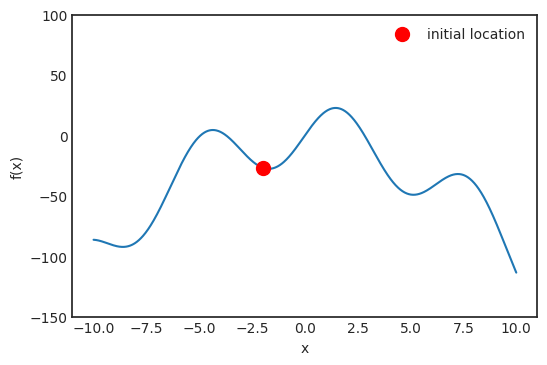If we want to find the peak, which direction should we go? Of course, we should go where the slope is increasing. The derivative of a function formalizes this concept. Mathematically, the derivative is defined byAlthough this quantity seems mysterious at first glance, it has a straightforward geometric meaning. Let's look at the function more closely around the point where we take the derivative.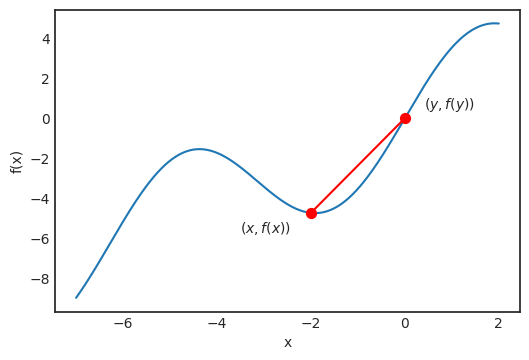For any x and y, the line passing through f(x) and f(y) is defined by the equationIn general, if we have any line defined by at + b for some a and b, the quantity a is called the slope of the line. This can be negative and positive as well. Lines with a positive slope go upward, while negative ones go downward. Higher values in absolute value mean steeper lines. If we let y closer and closer to x as it is in the definition of derivative, we see that the line becomes the tangent of the function graph at x.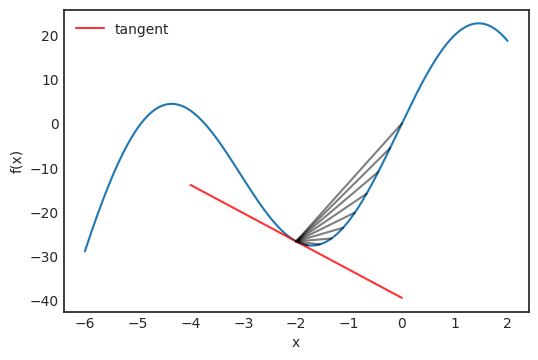The tangent is given by the functionand we can describe its direction with the vector (1, f'(x)).

If we imagine ourselves again in the position of a mountain climber starting from x₀ = -2.0, we should go in the direction where the tangent is rising. If the tangent slope is large, we would also like to take a large step, while if the slope is close to zero, we should take a smaller step to make sure we don't go over the peak. To formalize this mathematically, we should go to the next point defined bywhere λ is a parameter, setting how large the step should be in the right direction. This is called the learning rate. In general, subsequent steps are be defined byPositive derivative means that the tangent is increasing. Thus we want to go forward, while a negative derivative is a decreasing tangent, so we want to turn back. We can visualize this process.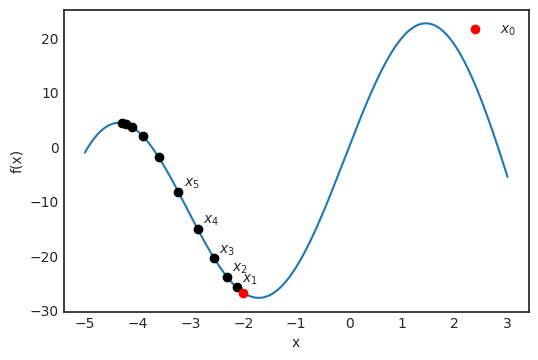As we can see, this simple algorithm successfully found a peak. However, this is not the global maximum of the function, which can be seen by looking at the image. To get a little ahead of ourselves, this is a potential issue for a broad family of optimizing algorithms, but there are solutions for it.

In this simple case, we have only maximized a function of a single variable. This is useful to illustrate the concept. However, in real-life scenarios, millions of variables can be present. For neural networks, this is definitely the case. In the next part, we will see how this simple algorithm can be generalized for optimizing multidimensional functions!

## Optimizing in multiple dimensions

For a single variable function, we could think about the derivative as the slope of the tangent line. However, for multiple variables, this is not the case. Let's try to build intuition first by looking at a concrete example! Define the functionwhich will be our toy example in this section.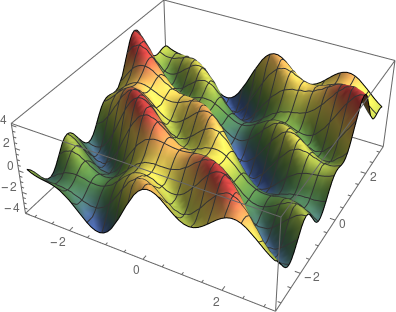For functions of two variables, the graph is a surface. We immediately see that the concept of the tangent line is not well defined since we have many tangent lines to a given point in the surface. In fact, we have a whole plane of them. This is called the tangent plane.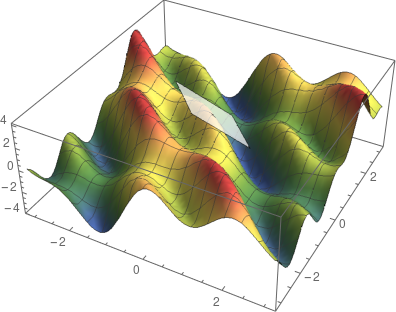However, this tangent plane contains two very special directions. Suppose that we are looking at the tangent plane at (0, 0). For every multivariable function, fixing all but one variable is a function of a single variable. In our case, we would haveandWe can visualize these functions by slicing the surface with a vertical plane perpendicular to one of the axes. Where the plane and the surface meet is the graph of f(x, 0) or f(0, y), depending on which plane you use. This is how it looks.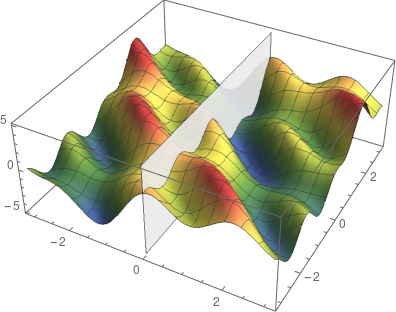For these functions, we can define the derivatives as we have done in the previous section. These are called partial derivatives and they play an essential role in generalizing our peak finding algorithm. To formalize it mathematically, they are defined byEach partial derivative represents a direction in our tangent plane. This is how we can visualize it.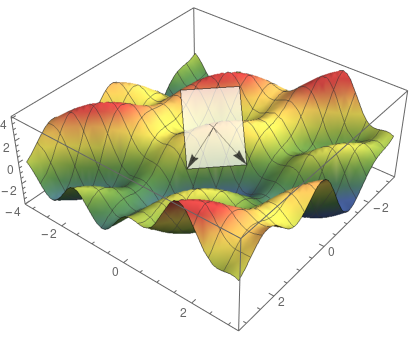The values of partial derivatives are the slopes of the special tangent lines. The direction of steepest ascent is given by the gradient, which is defined byNote that the gradient is a direction in the parameter space. The gradients can be visualized in the two-dimensional plane easily, which looks like the following in our case.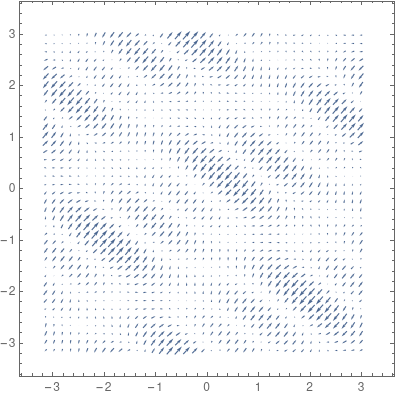To summarize, the peak finding algorithm is nowwhich is called gradient ascent. To find the minimum of a function, we take a step in the direction of the negative gradient, which is the direction of steepest descent:This version is called gradient descent. You have probably seen this one more frequently since we want to minimize the loss in machine learning.

### Why does the gradient point to the steepest ascent?

In this setting, it is not trivial why the gradient gives us the direction of the steepest ascent. To provide a precise explanation, we need to do some mathematics. Besides slicing the surface with vertical planes perpendicular to the x or y axis, we can slice it with a vertical plane given by any direction (a, b). With the partial derivatives, we hadWe can think about these as derivatives of f(x, y) along the directions (1, 0) and (0, 1). Although these directions are of special significance, we can do this in any direction. Say we have the directionthen the directional derivative with respect to this direction is defined byNote that the last identity is nothing else than the dot product (also called scalar or inner product) of the direction vector and the gradient, the same dot product you probably encountered in your high school geometry classes. So,The question is the following: which direction maximizes the directional derivative? This would be the direction of the steepest ascent, so if we want to optimize, we want to know this particular direction. To see that this is nothing else than the gradient itself, as we have mentioned, recall that we can write the dot product aswhere |.| denotes the length of a vector, and α is the angle between the two vectors. (This is true in an arbitrary number of dimensions, not just in two dimensions.) It is easy to see that this expression is maximized when cos α = 1, that is, α is zero. This means that the two vectors are parallel. Thus the direction of e must be the same as the gradient.

## Training neural networks

Now we are ready to move from theory to practice and see how we can train neural networks. Suppose that our task is to classify images n dimensional feature vectors into c classes. To mathematically formalize our situation, our neural network is represented by the function f, mapping the n-dimensional feature space to the c-dimensional space:The neural network itself is a parameterized function. For notational convenience, we can denote its parameters with a single m-dimensional vectorTo explicitly express dependence on parameters, it is customary to writeTraining a neural network is equivalent to finding the minimum of the loss functionmapping the space of neural network parameters to real numbers. The loss function takes the formwhere x-es are the data points with y-s as observations, and L is the termwise loss function. For instance, if J is the cross-entropy loss, thenwhereThis might seem innocent enough, but it can be challenging to compute. In real life, the number of data points N can be in the millions, not to say the number of parameters m. So, we have a sum with millions of terms, for which we need to calculate millions of derivatives to minimize. How can we solve this problem in practice?

To use gradient descent, we have to calculatewhere the expected value is taken with respect to the empirical probability distribution given our training data. We can treat the sequenceas independent, identically distributed random variables. According to the Law of Large Numbers,holds, where the limit expected value is taken with respect to the true underlying probability distribution of the data. (Which is unknown.) To elaborate more, this means that as we increase our training data, our loss function converges to the true loss. As a consequence, if we subsample our data and only calculate the gradientfor some i instead of all, we still obtain a reasonable estimate if we compute enough. This is called stochastic gradient descent or SGD in short.

In my opinion, there are three fundamental developments that enabled researchers and data scientists to effectively train deep neural networks: utilizing GPU-s as a general-purpose computing tool, backpropagation, and finally, stochastic gradient descent. Safe to say that without SGD, wide adoption of deep learning would not have been possible.

As with almost every new approach, SGD also introduces a whole new can of worms. The obvious question is, how large should our subsample size be? Too small size might result in a noisy gradient estimation, while too large has diminishing returns. Selecting the subsample also needs to happen with care. For example, if all the subsamples belong to one class, the estimate will probably be off by a mile. However, these issues can be solved in practice by experimentation and proper randomization of the data.

Gradient descent (with the SGD variant as well) suffers from several issues which can make them ineffective under some circumstances. For instance, as we have seen, the learning rate controls the step size we will take in the direction of the gradient. Generally, we can make two mistakes regarding this parameter. First, we can make the step too large, so the loss fails to converge and might even diverge. Second, we might never arrive at a local minimum because we go too slow if the step is too small. To demonstrate this issue, let's take a look at a simple example and study the f(x) = x + sin x function.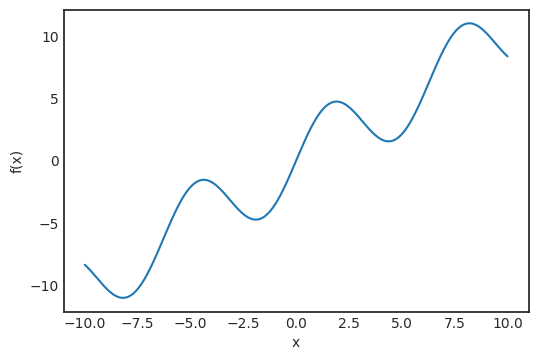Suppose that we start the gradient descent from x₀ = 2.5, with learning rates α = 1, α = 0.1 and α = 0.01.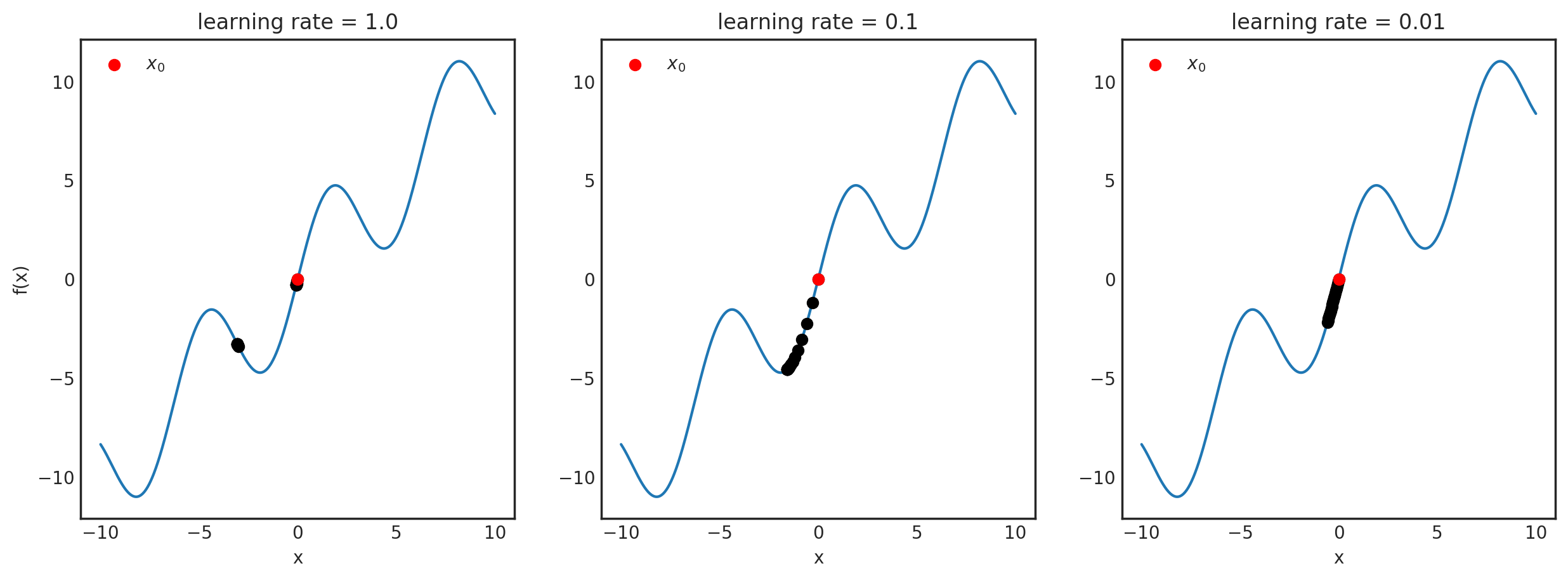It might not be obvious what is happening here, so let's plot the x-s for each learning rate.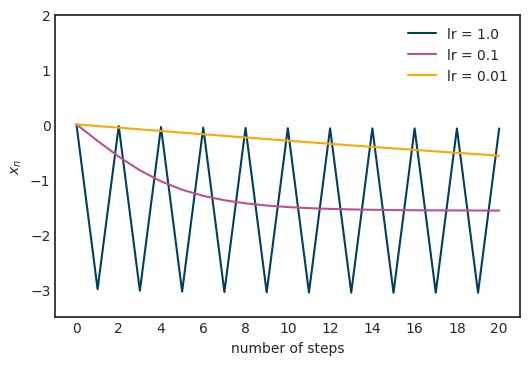For α = 1, the sequence is practically oscillating between two points, failing to converge to the local minimum, while for α = 0.01, the convergence seems to be very slow. In our concrete case, α = 0.1 seems just right. How do you determine this in a general setting? Their main idea here is that the learning rate does not necessarily have to be constant. Heuristically, if the magnitude of the gradient itself is large, we should reduce the learning rate to avoid jumping too far. On the other hand, if the magnitude is small, it probably means that we are getting close to a local optimum, so to avoid overshooting, the learning rate definitely shouldn't be increased. Algorithms changing the learning rate dynamically are called adaptive.

One of the most famous examples of such an adaptive algorithm is AdaGrad. It cumulatively stores gradient magnitude and scales the learning rate with respect to that. AdaGrad defines an accumulation variable r₀ = 0 and updates it with the rulewheredenotes the componentwise product of two vectors. This is then used to scale the learning rate:where δ is a small number for numerical stability, and the square root is taken componentwise. First, when the gradient is large, the accumulation variable grows rather fast, thus decreasing the learning rate. When the parameter is near a local minimum, gradients get smaller, so the learning rate stops practically.

Of course, AdaGrad is one possible solution to this problem. More and more advanced optimization algorithms are available every year, solving a wide range of issues related to gradient descent. However, experimenting with the learning rate and tuning it is beneficial even with the most advanced optimization methods.

Regarding issues with gradient descent, another is, for instance, to make sure that we find a global optimum or a local optimum close to it in value. As you can see in the previous example, gradient descent often gets stuck in a bad local optimum. To get a good picture about the solution for this and the other issues, I recommend reading through Chapter 8 of the Deep Learning textbook by Ian Goodfellow, Yoshua Bengio, and Aaron Courville.

## How does the loss function for deep neural networks look like?

In our examples during the previous sections, we have only visualized toy examples like f(x) = 25 sin x - x². There is a reason for this: plotting a function is not straightforward for more than two variables. Since our inherent limitations, we are only able to see and think in at most three dimensions. However, to grasp the difficulty of how the loss function of a neural network looks, we can employ several tricks. One excellent paper about this is Visualizing the Loss Landscape of Neural Nets by Hao Li et al., who were able to visualize the loss function by essentially choosing two random directions and plotting the two-variable function(To avoid distortions by scale invariance, they also introduced some normalizing factors for the random directions.) Their investigations revealed how skip connections in ResNet architectures shape the loss landscape, making it easier to optimize.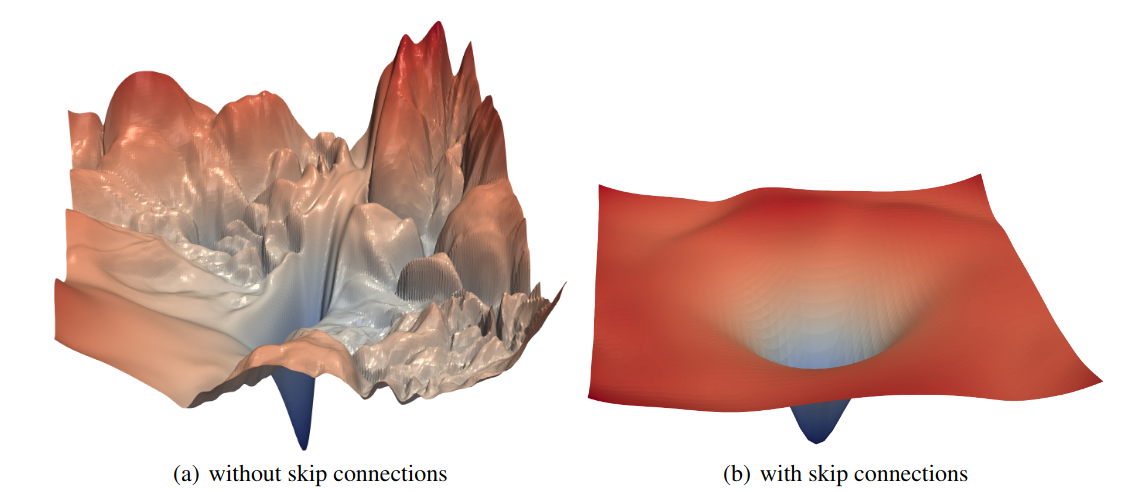Source: Visualizing the Loss Landscape of Neural Nets by Hao Li et al.

Regardless of the significant improvement made by skip connections, my point was to demonstrate that highly multidimensional optimization is hard. By looking at the first part of the figure, we see many local minima, sharp peaks, plateaus, and so on. Good architecture design can make the job of optimizers easier, but with thoughtful optimization practices, we can tackle more complicated loss landscapes. These go hand in hand.

## Conclusion

In the previous sections, we have learned the intuition behind gradients and defined them in a mathematically precise way. We have seen that for any differentiable function, no matter the number of variables, the gradient always points towards the steepest ascent, which is the foundation of the gradient descent algorithm. Although conceptually very simple, it has significant computational difficulties when applied to functions with millions of variables. This problem is alleviated by stochastic gradient descent. However, there are many more issues: getting stuck in a local optimum, selecting the learning rate, etc. Because of these issues, optimization is hard and requires attention from both researchers and practitioners. There is a very active community out there, making it constantly better, with amazing results! After understanding the mathematical foundations of optimization for deep learning, now you are on the right path to improve state-of-the-art! Some great papers to get you started: